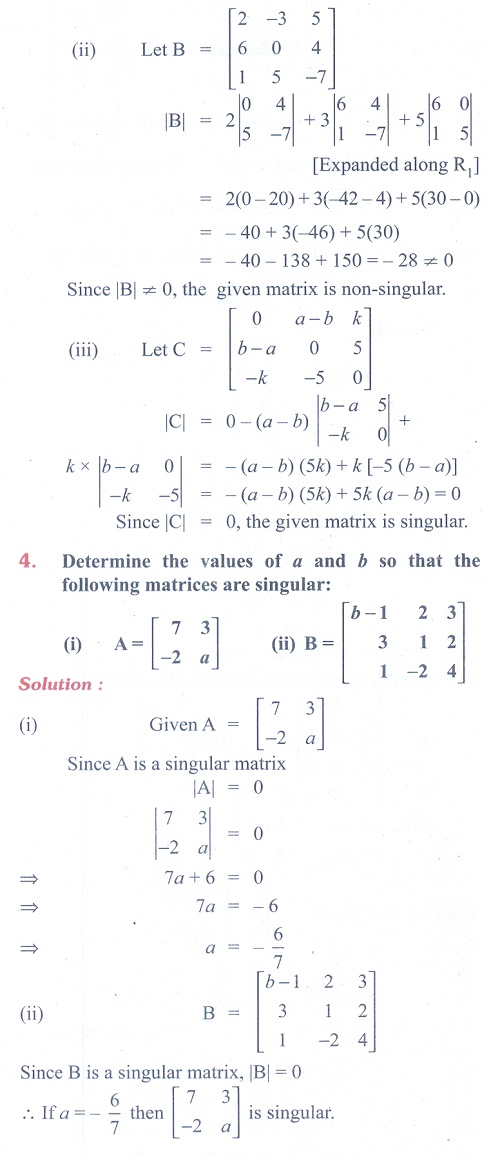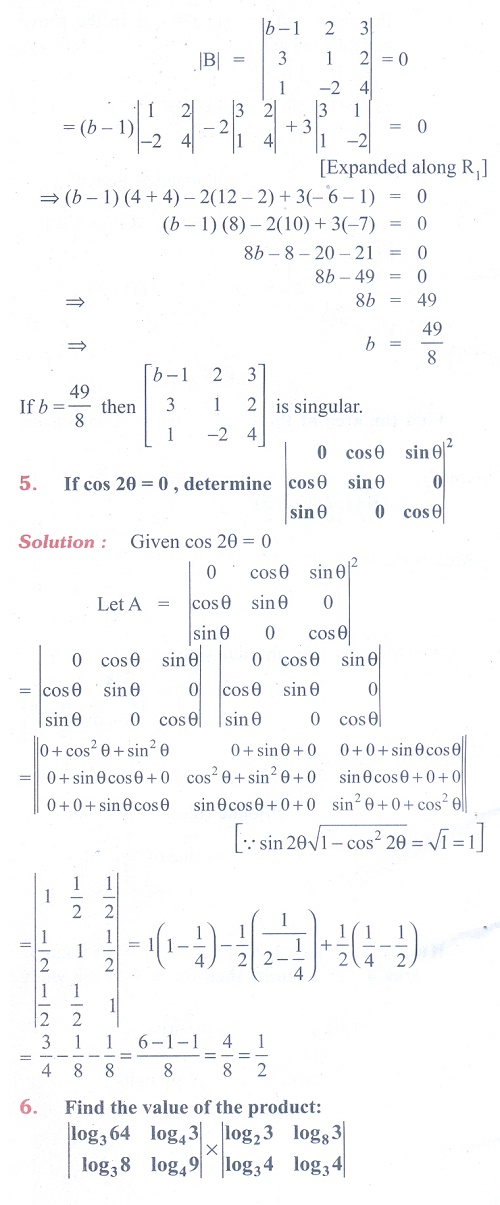Home | | Maths 11th std | Exercise 7.4: Determinants: Area of a Triangle, Singular and non-singular Matrices

# Exercise 7.4: Determinants: Area of a Triangle, Singular and non-singular Matrices

Maths Book back answers and solution for Exercise questions - Mathematics : Determinants: Area of a Triangle, Singular and non-singular MatricesTags : Problem Questions with Answer, Solution | Mathematics , 11th Mathematics : UNIT 7 : Matrices and Determinants
Study Material, Lecturing Notes, Assignment, Reference, Wiki description explanation, brief detail
11th Mathematics : UNIT 7 : Matrices and Determinants : Exercise 7.4: Determinants: Area of a Triangle, Singular and non-singular Matrices | Problem Questions with Answer, Solution | Mathematics